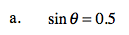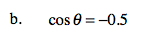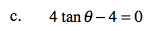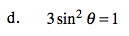### Home > A2C > Chapter 10 > Lesson 10.1.1 > Problem10-15

10-15.
1. Solve each equation for 0 ≤ θ ≤ 360. You may need your calculator, but remember that your calculator only gives one answer. Most of these problems have more than one answer. Think about all four quadrants. Homework Help ✎

1. sin θ = 0.5

2. cos θ = −0.5

3. 4 tan θ − 4 = 0

4. 3 sin2 θ = 1Remember the angles from special right triangles.

30° and ?120° and ?Simplify the equation first.This is not one of the special angles.
Use a calculator to find one answer.